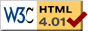### Journée-séminaire de combinatoire

#### (équipe CALIN du LIPN, université Paris-Nord, Villetaneuse)

Le 26 octobre 2021 à 16h15 en B107 (& visioconférence), Michael Drmota nous parlera de : Universal asymptotics for positive catalytic equations

Résumé : Catalytic equations appear in several combinatorial applications, most notably in the numeration of lattice path and in the enumeration of planar maps. The main purpose of this paper is to show that the asymptotic estimate for the coefficients of the solutions of (so-called) positive catalytic equations has a universal asymptotic behavior. In particular, this provides a rationale why the number of maps of size n in various planar map classes grows asymptotically like $c.n^{−5/2}\gamma^n$, for suitable positive constants $c$ and $\gamma$. Essentially we have to distinguish between linear catalytic equations (where the subexponential growth is $n^{−3/2}$) and non-linear catalytic equations (where we have $n^{−5/2}$ as in planar maps). Furthermore we provide a quite general central limit theorem for parameters that can be encoded by catalytic functional equations, even when they are not positive. Joint work with Marc Noy and Guan-Ru Yu.

[Slides.pdf] [arXiv] [vidéo]

 Dernière modification : Monday 24 January 2022Contact pour cette page : Cyril.Banderier at lipn.univ-paris13.fr